# LE CHATELIER'S PRINCIPLE

The le Chatelier's principle can be stated as:

When external stress is applied on a system at dynamic equilibrium, the system shifts the position of equilibrium so as to nullify the effect of stress.

Stress can be applied on chemical systems by changing the concentration or pressure or temperature. Thus it can be stated as:

When a chemical system at dynamic equilibrium is disturbed by changing the concentration of either reactants or products; or by changing the partial pressures of any of gaseous reactants or of gaseous products; or temperature, the position of equilibrium is changed in that direction so as to establish a new equilibrium state i.e., either forward reaction or backward reaction is favored.

1) Explanation

2) Effect of concentration

3) Effect of pressure

4) Effect of temperature

5) Illustrations

6) Industrial applications

7) Test your understanding - MCQ

## EXPLANATION OF LE CHATELIER'S PRINCIPLE

### EFFECT OF CHANGE IN CONCENTRATION

The change in concentration can affect gaseous systems or liquid solution systems only. However this does not affect the solid and pure liquid systems since their active masses are always taken as unity.

By using le Chatelier's principle, the effect of change in concentration on systems at equilibrium can be explained as follows:

1) When the concentration of reactant(s) is increased, the system tries to reduce their concentration by favoring the forward reaction.

2) When the concentration of product(s) is increased, the system tries to reduce their concentration by favoring the backward reaction.

3) When the concentration of reactant(s) is decreased, the system tries to increase their concentration by favoring the backward reaction.

4) When the concentration of product(s) is decreased, the system tries to increase their concentration by favoring the forward reaction.

When the concentration of reactants is increased, the number of effective collisions between them increases which in turn increases the rate of forward reaction. Thus the forward reaction is more favored over the backward reaction until the new equilibrium is established. At this new equilibrium, the rates of both forward and backward reactions become equal again and the reaction quotient becomes approximately equal to the equilibrium constant.

Remember that small changes in concentration do not affect the equilibrium constant appreciably.

Illustration:

The decomposition of gaseous PCl5 is a reversible reaction.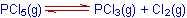Let the equilibrium concentrations of PCl5, PCl3 and Cl2 are respectively [PCl5], [PCl3] and [Cl2]. The KC for this reaction can be written as:Also we know that at equilibrium KC = Q.

Let the concentration of PCl5 is doubled to disturb the equilibrium. This will change the reaction quotient, Q to:After disturbing the equilibrium, the value of Q becomes less than KC. In order to restore the Q value to KC,  the concentration of PCl5 must be decreased while the concentrations of PCl3 and Cl2 are to be increased. This is achieved by favoring the forward reaction.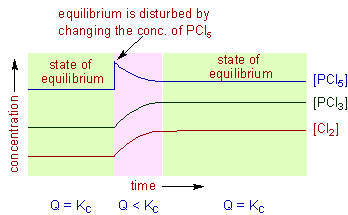The forward reaction is also favored by removing the products from the reaction mixture (decrease in the concentration of products). Upon removal of products, the rate of forward reaction becomes greater than that of backward reaction momentarily. This will also decrease the reaction quotient. Hence the system tries to reestablish the equilibrium by converting more reactants to products so as to make the rates of both forward and backward reactions become equal again.

For example, in case of the decomposition of PCl5, if the concentration of Cl2 is increased by two times at equilibrium, the Q value becomes greater than the KC value.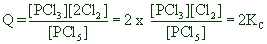Hence the system tries to restore the value of Q to KC again. The backward reaction is favored to decrease the concentration of Cl2. However the concentration of PCl5 also decreases automatically while the concentration of PCl5 increases while doing so.### EFFECT OF CHANGE IN PRESSURE

The change in pressure only affects the equilibrium of systems involving at least one gas. The le Chatelier's principle can be applied to understand the effect of change in pressure on the systems at equilibrium as follows.

1) When the partial pressure of any of the gaseous reactants or of the products is increased, the position of equilibrium is shifted so as to decrease its partial pressure. This is usually achieved by favoring the reaction in which there is decrease in the number of moles of gaseous components.

2) When the partial pressure of any of the gaseous reactants or of the products is decreased, the position of equilibrium is shifted so as to increase its partial pressure. This can be achieved by favoring that reaction in which there is increase in the number of moles of gaseous components.

However, it is not always correct to say that the equilibrium is shifted whenever there is a change in the total pressure of the system. The equilibrium is not always disturbed upon changing the pressure of the entire system. It is only disturbed whenever there is a change in the partial pressure of any or all of the gaseous reactants or products in the equilibrium for which  Δng ≠ 0.

Where Δng = (no. of moles of gaseous products) - (no.of moles of gaseous reactants)

Strictly speaking, the equilibrium is only shifted when the ratio of product of partial pressures of products to the product of partial pressures of reactants i.e., the reaction quotient in terms of partial pressures, Qp is disturbed. The position of equilibrium is shifted so as to make Qp become equal to the value of Kp again.

The Qp can be changed in the following cases:

1) By adding or removing any gaseous reactant or product at constant volume. The effect is same as changing the concentration as explained above.

2) By changing the volume of the system (or in other words by changing the pressure of entire system) at equilibrium for which the Δng ≠ 0. In this case, however, the pressure of the entire system is also changed.

Illustration:

For the decomposition of PCl5, the Kp can be written as: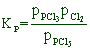For this reaction Δng ≠ 0; i.e., Δng = (1+1)-(1) = 1

Hence for this reaction, if the pressure of the system is increased by 2 times by halving the volume, the reaction quotient, Qp is changed to:In order to restore back the Qp value again to Kp, the denominator value i.e., the partial pressure of PCl5 must be increased. This is only achieved by favoring the forward reaction in which less number of gaseous products are formed. i.e., Two moles of products (PCl3 and Cl2) are converted to one mole of reactant (PCl5)

Therefore we can say, at least in this case, if the pressure of the system is increased, the system tries to decrease it by favoring the reaction in the direction of decreasing the number of moles of gaseous components.

3) By adding a non reacting inert gas to the system at constant pressure when Δng ≠ 0: The addition of an inert gas at constant pressure increases the volume of the system. Hence the partial pressures of gaseous components are decreased. This will disturb the Qp value.  Hence the system tries to restore the Qp to Kp.

Whereas, the Qp value cannot be changed in the following cases:

1) By changing the volume of the system (or in other words by changing the pressure of entire system) at equilibrium for which Δng = 0. Hence the position of equilibrium is not going to be changed.

Illustration:

Let us consider the decomposition of HI to H2 and I2.For this reaction, the Δng = (1+1) - (2) = 0.

The Kp can be written for this equilibrium as: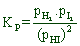If the volume of the system is halved to double the pressure, the Qp value is not changed as illustrated below.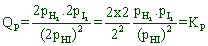In this case, the increase in the product of partial pressures of products (the numerator value) is nullified by the increase in the product of partial pressures of reactants (the denominator value).

Therefore, when Δng = 0, there is no effect of changing the pressure of entire system.

However, remember that if the partial pressures of gaseous components are changed to different extent, the equilibrium is disturbed even if Δng = 0.

2) By adding a non reacting inert gas to the system at constant volume. In this case, the pressure of the entire system is increased. However the individual partial pressures of gases participating in the reaction are not changed since the volume is not changed. Hence the Qp value does not change. That is why there will be no effect of adding an inert gas to the system at constant volume.

3) By adding a non reacting inert gas to the system at constant pressure when Δng = 0 . The volume of the system is increased when a non reacting gas is added to the system at constant pressure. This will decrease the partial pressures of each gaseous component. However the Qp value is not disturbed since the decrease in the numerator value is cancelled by the decrease in the denominator value.

However any reacting gas can disturb the equilibrium.

### EFFECT OF CHANGE IN TEMPERATURE

The effect of temperature can be understood by using le Chatelier's principle as follows:

1) Increase in the temperature of the system favors the endothermic reaction.

The increase in temperature increases the amount of heat in the system. Hence it tries to remove the excess of heat by favoring that reaction in which heat is absorbed i.e., the endothermic reaction.

2) Decrease in the temperature of the system favors the exothermic reaction.

In this case, the temperature is decreased by removing the heat content from the system. Hence the system tries to restore the temperature back by favoring the exothermic reaction i.e., the reaction in which the heat is liberated.

It is very important to note that, during the change in temperature, the system establishes a new equilibrium for which the value of equilibrium constant is different from the original constant i.e., the equilibrium constant depends on the temperature.

Illustration:

Consider the following exothermic reversible reaction:In general, when we say a reaction is exothermic, the forward reaction is exothermic whereas, the backward reaction is endothermic.

This reaction can also be written as;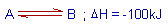The negative sign of ΔH indicates the exothermic nature of the forward reaction.

It is quite helpful to consider the 'heat' as one of the product of the reaction.

The equilibrium constant, KC can be written as:If the equilibrium is disturbed by increasing the temperature by adding heat,  the endothermic backward reaction is favored to remove the heat from the system. While doing so, the concentration of 'B' decreases and a new equilibrium position is established for which the new equilibrium constant, K'C can be written as:Where K'C < KC since [B'] < [B] and [A'] > [A]

If the temperature is decreased by removing the heat from the system, the system tries to increase the heat content by favoring the exothermic forward reaction. In this case, the new equilibrium constant, let us say K"C is greater than the KC.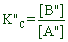Where K'C > KC since [B"] > [B] and [A"] < [A]

### EFFECT OF CATALYST

A catalyst has no effect on the position of the equilibrium since it increases not only the rate of forward reaction but also the rate of backward reaction. However it does help the system to reach the equilibrium faster.

## ILLUSTRATIONS OF LE CHATELIER'S PRINCIPLE

The CoCl2.6H2O or [Co(H2O)6]Cl2 is a deep purple colored solid. It forms a purple colored solution when is dissolved in water. The color of [Co(H2O)6]2+ ion is pink. However it exists in equilibrium with small amount of [CoCl4]2- that is intense blue in color. The equilibrium is shown below.  The purple color of the solution is the result of combination of these two colors.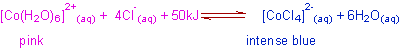The purple color of the aqueous solution of Cobalt(II) chloride can be changed by adjusting different variables in the following experiments.

1) The color of the solution turns intense blue upon addition of conc. HCl to the first test tube.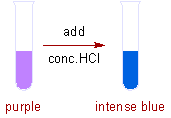This is because of increase in the concentration of Cl- ions, which are furnished by HCl. As a result, the forward reaction is favored to give more [CoCl4]2-. Hence the color is turned to intense blue.

The Cl- ions are common to both HCl and [Co(H2O)6]Cl2. Hence this is also referred to as "common ion effect".

2) The color of the solution turns to pale pink by adding excess of water in the second experiment.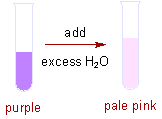According to le Chatelier's principle, the backward reaction is favored when the concentration of one of the product increases. Hence increase in the concentration of water favors backward reaction. That will result in the formation of more [Co(H2O)6]2+, which is pale pink in color.

3) In the third experiment, the color turns to blue upon heating the purple colored solution.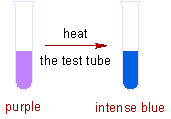According to le Chatelier's principle, when heat is added to the system, the endothermic reaction is favored to remove heat from the system. In this case, the formation of [CoCl4]2- is an endothermic reaction. Hence more amount of blue colored [CoCl4]2- is formed.

Note: The intense blue color of [CoCl4]2- is due to its tetrahedral geometry. You will learn this in more detail at advanced level of inorganic chemistry.

Try your self to answer the question: What will happen to the color when small amount of aqueous solution of AgNO3 is added to the first test tube?

## INDUSTRIAL APPLICATIONS OF LE CHATELIER'S PRINCIPLE

### HABER PROCESS

In Haber process, the ammonia  is synthesized by combining pure nitrogen and hydrogen gases in 1:3 ratio in presence of finely powdered iron catalyst and molybdenum promoter at around 450oC and at about 250 atm. of pressure.The le Chatelier's principle helps in choosing these conditions to improve the yields of ammonia as explained as below.

Effect of pressure: In the forward reaction (synthesis of ammonia), the number of moles of gaseous components is decreasing.

i.e., Δng = (2) - (1+3) = -2

Hence the synthesis of ammonia is favored by increasing the pressure of the system. Industrially, 100 - 250 atm. of pressure is employed.

Effect of temperature: Since the forward reaction is exothermic, the increase in temperature favors the backward reaction i.e., the dissociation of ammonia. That means according to le Chatelier's principle, the synthesis of ammonia is favored at lower temperatures. However the reaction will be too slow at lower temperatures (a kinetic restriction). Hence this reaction is carried out at optimal temperatures i.e., at about 450 - 550 oC to overcome the kinetic barrier.

Removal of ammonia: The forward reaction can also be favored by removing ammonia from the system from time to time by liquefying it.

Catalyst: To increase the speed of the reaction, finely powdered or porous iron is used as catalyst. Its efficiency can be improved by adding molybdenum or oxides of potassium and aluminium.

### CONTACT PROCESS

In the contact process, sulfuric acid, the king of chemicals, is manufactured on large scale. The major steps involved in the process are: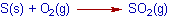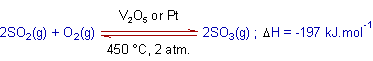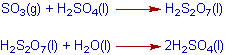The crucial step is the oxidation of sulfur dioxide, SO2 to sulfur trioxide, SO3. It is a reversible reaction. At normal conditions, the equilibrium lies far to the left and the amount of sulfur trioxide formed is very small. To improve the yield of sulfur trioxide, the reaction is carried out at around 450oC and 2 atm pressure in presence of V2O5 or Pt, which acts as a catalysts.

These conditions are chosen by applying le Chatelier's principle as explained below.

Effect of pressure: In the forward reaction (formation of sulfur trioxide), the number of moles of gaseous components is decreasing.

i.e., Δng = (2) - (2+1) = -1

Hence the forward reaction is favored by increasing the pressure of the system. However, at high pressures, the iron towers used in the contact process are corroded. Hence the process is carried out at optimal pressures like 2 atm.

Effect of temperature: Since the forward reaction is exothermic, at higher temperatures the backward reaction i.e., the dissociation of sulfur dioxide is more favored. However the reaction will be too slow at lower temperatures. Hence this reaction is carried out at optimal temperatures i.e., around 450 oC.

Catalyst: To increase the speed of the reaction, V2O5 or Pt are used as catalysts.